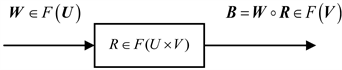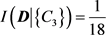﻿ 配电变压器状态评价权重系数改进方法的研究

# 配电变压器状态评价权重系数改进方法的研究Method for Evaluating State of Distribution Transformer Based on Fuzzy Theory

Abstract: With the continuous development of the economy and society, the demand for electricity in all as-pects of society is gradually expanding, which requires the power system to be more secure and re-liable. In this paper, the related theoretical knowledge related to the state evaluation of distribution transformers is deeply studied. The fuzzy comprehensive evaluation method is introduced, and the general algorithm and improved algorithm for determining the weight coefficients are analyzed. The weight coefficients of the two algorithms are calculated by an example. It is concluded that the calculation of the state quantity weight coefficient in the distribution transformer state evaluation should be improved based on the rough set theory based weight coefficient determination method.

1. 引言

2. 模糊综合评判方法

2.1. 模糊集合的基本概念

2.2. 模糊综合评判的步骤

$B=W\circ R=W\circ \left[\begin{array}{c}{W}_{1}\circ {R}_{1}\\ {W}_{2}\circ {R}_{2}\\ ⋮\\ {W}_{n}\circ {R}_{n}\end{array}\right]=W\circ \left[\begin{array}{c}{B}_{1}\\ {B}_{2}\\ ⋮\\ {B}_{n}\end{array}\right]$ (1.1)Figure 1. Fuzzy converter

1) 确定被评价的对象X。这里表示为需要评价的配电网某变压器。

2) 建立因素集U，也就是评价指标体系。

3) 建立表示评价结果等级的评价集V。

${v}_{i}$ 为评价等级，等级数为m，则评价集 $V=\left\{{v}_{1},{v}_{2},\cdots ,{v}_{m}\right\}$ 。本文中将配电变压器的运行状态和状态

$V=\left({v}_{1},{v}_{2},{v}_{3},{v}_{4}\right)=\left(正常,注意,异常,严重\right)$ (1.2)

4) 确定权重系数集W

$\underset{i=1}{\overset{n}{\sum }}{w}_{i}=1$ (1.3)

3. 基于粗糙集理论的权重系数确定方法的研究

3.1. 状态量权重系数的确定方法分类

3.2. 基于粗糙集理论权重系数的确定方法

3.2.1. 粗糙集理论确定权重系数的一般算法

1) 决策表的建立

2) 依赖度的计算

${\gamma }_{C}\left(D\right)=\frac{|po{s}_{C}\left(D\right)|}{|U|}$ (2.1)

${\gamma }_{C-{C}_{i}}\left(D\right)=\frac{|po{s}_{C-{C}_{i}}\left(D\right)|}{|U|}$ (2.2)

3) 重要度的计算

$sig\left({C}_{i}\right)={\gamma }_{C}\left(D\right)-{\gamma }_{C-{C}_{i}}\left(D\right)$ (2.3)

4) 权重系数的计算

$W\left({C}_{i}\right)=\frac{sig\left({C}_{i}\right)}{\underset{i=1}{\overset{m}{\sum }}sig\left({C}_{i}\right)}$ (2.4)

3.2.2. 改进后的权重系数的确定算法

1) 条件熵的计算

$I\left(D|C\right)=\underset{i=1}{\overset{m}{\sum }}\frac{{|{C}_{i}|}^{2}}{{|U|}^{2}}\underset{j=1}{\overset{m}{\sum }}\frac{|{D}_{j}\cap {C}_{i}|}{|{C}_{i}|}\left(1-\frac{|{D}_{j}\cap {C}_{i}|}{|{C}_{i}|}\right)$ (2.5)

2) 改进后重要度的计算

$sig\left({C}_{i}\right)=I\left(D|\left(C-{C}_{i}\right)\right)-I\left(D|C\right)+\frac{\underset{a\in C}{\sum }|a\left(x\right)|-\underset{a\in \left(C-{C}_{i}\right)}{\sum }|a\left(x\right)|}{\underset{a\in C}{\sum }|a\left(x\right)|}$ (2.6)

3) 改进后的权重系数的计算

$W\left({C}_{i}\right)=\frac{sig\left({C}_{i}\right)+I\left(D|\left\{{C}_{i}\right\}\right)}{\underset{a\in C}{\sum }\left\{sig\left(a\right)+I\left(D|\left\{a\right\}\right)\right\}}$ (2.7)

4. 实例分析Table 1. Oil insulation fault diagnosis decision table

4.1. 决策表的知识分类

$U/IND\left({C}_{1}\right)=\left\{\left\{1,2,3\right\},\left\{4,5,6\right\}\right\}$

$U/IND\left({C}_{2}\right)=\left\{\left\{1,2,3,4,6\right\},\left\{5\right\}\right\}$

$U/IND\left({C}_{3}\right)=\left\{\left\{1,4\right\},\left\{2,5\right\},\left\{3,6\right\}\right\}$

$U/IND\left(C\right)=\left\{\left\{1\right\},\left\{2\right\},\left\{3\right\},\left\{4\right\},\left\{5\right\},\left\{6\right\}\right\}$

$U/IND\left(D\right)=\left\{\left\{1,4,5\right\},\left\{2,3,6\right\}\right\}$

$po{s}_{C}\left(D\right)=\left\{1\right\}\cup \left\{2\right\}\cup \cdots \cup \left\{6\right\}=\left\{1,2,\cdots ,6\right\}=U$

4.2. 基于一般算法确定权重系数

$U/IND\left(C-{C}_{1}\right)=\left\{\left\{1,4\right\},\left\{2\right\},\left\{3,6\right\},\left\{5\right\}\right\}$

$U/IND\left(C-{C}_{2}\right)=\left\{\left\{1\right\},\left\{2\right\},\left\{3\right\},\left\{4\right\},\left\{5\right\},\left\{6\right\}\right\}$

$U/IND\left(C-{C}_{3}\right)=\left\{\left\{1,2,3\right\},\left\{4,6\right\},\left\{5\right\}\right\}$

${\gamma }_{C}\left(D\right)=\frac{|po{s}_{C}\left(D\right)|}{|U|}=\frac{|U|}{|U|}=\frac{6}{6}=1$

$po{s}_{C-{C}_{1}}\left(D\right)=\left\{1,2,3,4,5,6\right\}=U$

$po{s}_{C-{C}_{2}}\left(D\right)=\left\{1,2,3,4,5,6\right\}=U$

$po{s}_{C-{C}_{3}}\left(D\right)=\left\{5\right\}$

${\gamma }_{C-{C}_{1}}\left(D\right)=\frac{|po{s}_{C-{C}_{1}}\left(D\right)|}{|U|}=\frac{|U|}{|U|}=1$

${\gamma }_{C-{C}_{2}}\left(D\right)=\frac{|po{s}_{C-{C}_{2}}\left(D\right)|}{|U|}=\frac{|U|}{|U|}=1$

${\gamma }_{C-{C}_{3}}\left(D\right)=\frac{|po{s}_{C-{C}_{3}}\left(D\right)|}{|U|}=\frac{1}{6}$

$sig\left({C}_{1}\right)={\gamma }_{C}\left(D\right)-{\gamma }_{C-{C}_{1}}\left(D\right)=0$

$sig\left({C}_{2}\right)={\gamma }_{C}\left(D\right)-{\gamma }_{C-{C}_{2}}\left(D\right)=0$

$sig\left({C}_{3}\right)={\gamma }_{C}\left(D\right)-{\gamma }_{C-{C}_{3}}\left(D\right)=1-\frac{1}{6}=\frac{5}{6}$

$W\left({C}_{1}\right)=\frac{sig\left({C}_{1}\right)}{sig\left({C}_{1}\right)+sig\left({C}_{2}\right)+sig\left({C}_{3}\right)}=0$

$W\left({C}_{2}\right)=\frac{sig\left({C}_{2}\right)}{sig\left({C}_{1}\right)+sig\left({C}_{2}\right)+sig\left({C}_{3}\right)}=0$

$W\left({C}_{3}\right)=\frac{sig\left({C}_{3}\right)}{sig\left({C}_{1}\right)+sig\left({C}_{2}\right)+sig\left({C}_{3}\right)}=1$

4.3. 基于改进算法确定权重系数

$\begin{array}{c}I\left(D|C\right)=\underset{i=1}{\overset{m}{\sum }}\frac{{|{C}_{i}|}^{2}}{{|U|}^{2}}\underset{j=1}{\overset{m}{\sum }}\frac{|{D}_{j}\cap {C}_{i}|}{|{C}_{i}|}\left(1-\frac{|{D}_{j}\cap {C}_{i}|}{|{C}_{i}|}\right)\\ ={\left(\frac{1}{6}\right)}^{2}\left[\frac{1}{1}\left(1-\frac{1}{1}\right)+\frac{0}{1}\left(1-\frac{0}{1}\right)\right]+{\left(\frac{1}{6}\right)}^{2}\left[\frac{0}{1}\left(1-\frac{0}{1}\right)+\frac{1}{1}\left(1-\frac{1}{1}\right)\right]\\ \text{\hspace{0.17em}}\text{\hspace{0.17em}}+{\left(\frac{1}{6}\right)}^{2}\left[\frac{0}{1}\left(1-\frac{0}{1}\right)+\frac{1}{1}\left(1-\frac{1}{1}\right)\right]+{\left(\frac{1}{6}\right)}^{2}\left[\frac{1}{1}\left(1-\frac{1}{1}\right)+\frac{0}{1}\left(1-\frac{0}{1}\right)\right]\\ \text{\hspace{0.17em}}\text{\hspace{0.17em}}+{\left(\frac{1}{6}\right)}^{2}\left[\frac{1}{1}\left(1-\frac{1}{1}\right)+\frac{0}{1}\left(1-\frac{0}{1}\right)\right]+{\left(\frac{1}{6}\right)}^{2}\left[\frac{0}{1}\left(1-\frac{0}{1}\right)+\frac{1}{1}\left(1-\frac{1}{1}\right)\right]\\ =0\end{array}$

$\begin{array}{c}I\left(D|\left\{{C}_{1}\right\}\right)=\underset{i=1}{\overset{m}{\sum }}\frac{{|{C}_{i}|}^{2}}{{|U|}^{2}}\underset{j=1}{\overset{m}{\sum }}\frac{|{D}_{j}\cap {C}_{i}|}{|{C}_{i}|}\left(1-\frac{|{D}_{j}\cap {C}_{i}|}{|{C}_{i}|}\right)\\ ={\left(\frac{3}{6}\right)}^{2}\left[\frac{1}{3}\left(1-\frac{1}{3}\right)+\frac{2}{3}\left(1-\frac{2}{3}\right)\right]+{\left(\frac{3}{6}\right)}^{2}\left[\frac{2}{3}\left(1-\frac{2}{3}\right)+\frac{1}{3}\left(1-\frac{1}{3}\right)\right]\\ =\frac{2}{9}\end{array}$

$\begin{array}{c}I\left(D|\left(C-\left\{{C}_{1}\right\}\right)\right)=\underset{i=1}{\overset{m}{\sum }}\frac{{|{C}_{i}|}^{2}}{{|U|}^{2}}\underset{j=1}{\overset{m}{\sum }}\frac{|{D}_{j}\cap {C}_{i}|}{|{C}_{i}|}\left(1-\frac{|{D}_{j}\cap {C}_{i}|}{|{C}_{i}|}\right)\\ ={\left(\frac{2}{6}\right)}^{2}\left[\frac{2}{2}\left(1-\frac{2}{2}\right)+\frac{0}{2}\left(1-\frac{0}{2}\right)\right]+{\left(\frac{1}{6}\right)}^{2}\left[\frac{0}{1}\left(1-\frac{0}{1}\right)+\frac{1}{1}\left(1-\frac{1}{1}\right)\right]\\ \text{\hspace{0.17em}}\text{\hspace{0.17em}}\text{ }+{\left(\frac{2}{6}\right)}^{2}\left[\frac{0}{2}\left(1-\frac{0}{2}\right)+\frac{2}{2}\left(1-\frac{2}{2}\right)\right]+{\left(\frac{1}{6}\right)}^{2}\left[\frac{1}{1}\left(1-\frac{1}{1}\right)+\frac{0}{1}\left(1-\frac{0}{1}\right)\right]\\ =0\end{array}$

$I\left(D|\left\{{C}_{2}\right\}\right)=\frac{1}{3}$$I\left(D|\left(C-\left\{{C}_{2}\right\}\right)\right)=0$

$I\left(D|\left(C-\left\{{C}_{3}\right\}\right)\right)=\frac{1}{6}$

$\frac{\underset{a\in C}{\sum }|a\left(x\right)|-\underset{a\in \left(C-\left\{{C}_{i}\right\}\right)}{\sum }|a\left(x\right)|}{\underset{a\in C}{\sum }|a\left(x\right)|}=\frac{1}{3}$

$sig\left({C}_{1}\right)=I\left(D|\left(C-\left\{{C}_{1}\right\}\right)\right)-I\left(D|C\right)+\frac{\underset{a\in C}{\sum }|a\left(x\right)|-\underset{a\in \left(C-\left\{{C}_{i}\right\}\right)}{\sum }|a\left(x\right)|}{\underset{a\in C}{\sum }|a\left(x\right)|}=\frac{1}{3}$$sig\left({C}_{3}\right)=I\left(D|\left(C-\left\{{C}_{3}\right\}\right)\right)-I\left(D|C\right)+\frac{\underset{a\in C}{\sum }|a\left(x\right)|-\underset{a\in \left(C-\left\{{C}_{i}\right\}\right)}{\sum }|a\left(x\right)|}{\underset{a\in C}{\sum }|a\left(x\right)|}=\frac{1}{2}$

$W\left({C}_{1}\right)=\frac{sig\left({C}_{1}\right)+I\left(D|\left\{{C}_{1}\right\}\right)}{\underset{a\in C}{\sum }\left\{sig\left(a\right)+I\left(D|\left\{a\right\}\right)\right\}}=\frac{5}{16}=0.3125$

$W\left({C}_{2}\right)=\frac{sig\left({C}_{2}\right)+I\left(D|\left\{{C}_{2}\right\}\right)}{\underset{a\in C}{\sum }\left\{sig\left(a\right)+I\left(D|\left\{a\right\}\right)\right\}}=\frac{3}{8}=0.375$

$W\left({C}_{3}\right)=\frac{sig\left({C}_{3}\right)+I\left(D|\left\{{C}_{3}\right\}\right)}{\underset{a\in C}{\sum }\left\{sig\left(a\right)+I\left(D|\left\{a\right\}\right)\right\}}=\frac{5}{16}=0.3125$

4.4. 对比分析

5. 总结

 叶珍. 基于AHP的模糊综合评价方法研究及应用[D]: [硕士学位论文]. 广州: 华南理工大学, 2010.

 冯谦. 基于AHP-模糊综合评定模型在商业房地产评估中的运用[D]: [硕士学位论文]. 重庆: 重庆理工大学, 2019.

 曹杨敏. 用于药企环境管理绩效评价的AHP-模糊综合评价法模型构建与应用研究[D]: [硕士学位论文]. 杭州: 浙江工业大学, 2019.

 张娟. 基于AHP-模糊综合评价法的儿童安全座椅舒适性评价研究[D]: [硕士学位论文]. 杭州: 浙江工业大学, 2018.

 胡娱欧. 基于改进的模糊层次分析法的输电网规划综合评价研究[D]: [硕士学位论文]. 北京: 华北电力大学, 2016.

 刘杨. 基于SG-MA-ISPA模型的区域可持续发展评价研究[D]: [博士学位论文]. 重庆: 重庆大学, 2012.

 卢文达. 基于模糊层次分析法的状态评估系统的研究[D]: [硕士学位论文]. 北京: 华北电力大学, 2016.

 Zhang, Y., Wang, S. and Han, X. (2013) Re-search on Decision-Making Process of Condition-Based Maintenance. International Conference on Quality, Reliability, Risk, Maintenance, and Safety Engineering, Chengdu, 15-18 July 2013, 1437-1440.
https://doi.org/10.1109/QR2MSE.2013.6625840

 程瑞. 一种基于粗糙集和VPRS的第四方物流多因素评价方法[J]. 中国物流与采购, 2019(6): 43-44.

 王长忠, 陈德刚. 基于粗糙集的知识获取理论与方法[M]. 哈尔滨: 哈尔滨工业大学出版, 2010: 18.

 魏玲, 张琬林, 李阳. 基于模糊包含度的贝叶斯粗糙集模型[J]. 统计与决策, 2019, 35(2): 34-38.

 鲍新中, 刘澄. 一种基于粗糙集的权重确定方法[J]. 管理学报, 2009, 6(6): 729-732.

 Maity, A., Zaremby, D., Mcmullen, D., et al. (2011) Automated Scheduling Using Condition Based Maintenance. IEEE Conference on Prognostics and Health Management, Montreal, 20-23 June 2011, 7-11.
https://doi.org/10.1109/ICPHM.2011.6024331

 黄肖为, 吴健儿, 赵明娄, 等. 基于改进模糊层次分析法的电缆绝缘寿命评估模型[J]. 电气自动化, 2019, 41(4): 107-110.

 苗夺谦, 李道国. 粗糙集理论、算法与应用[M]. 北京: 清华大学出版社, 2008: 25-30.

Top